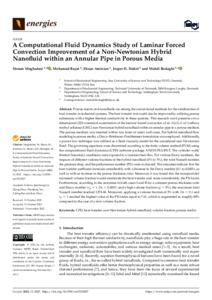# A computational fluid dynamics study of laminar forced convection improvement of a non-Newtonian hybrid nanofluid within an annular pipe in porous media

Moghadasi, H., Bayat, M., Aminian, E., Hattel, J.H. and , 2022. A computational fluid dynamics study of laminar forced convection improvement of a non-Newtonian hybrid nanofluid within an annular pipe in porous media. Energies, 15 (21): 8207.Preview
Text
1619746_Bodaghi.pdf - Published version

## Abstract

Porous inserts and nanofluids are among the conventional methods for the amelioration of heat transfer in industrial systems. The heat transfer rate could also be improved by utilizing porous substances with a higher thermal conductivity in these systems. This research work presents a two-dimensional (2D) numerical examination of the laminar forced convection of an Al2O3-CuO-carboxy methyl cellulose (CMC) non-Newtonian hybrid nanofluid within an annular pipe in a porous medium. The porous medium was inserted within two inner or outer wall cases. For hybrid nanofluid flow modeling in porous media, a Darcy–Brinkman–Forchheimer formulation was employed. Additionally, a power-law technique was utilized as a fluid viscosity model for the considered non-Newtonian fluid. The governing equations were discretized according to the finite volume method (FVM) using the computational fluid dynamics (CFD) software package ANSYS-FLUENT. The cylinder walls’ thermal boundary conditions were exposed to a constant heat flux. For various Darcy numbers, the impacts of different volume fractions of the hybrid nanofluid (0% to 5%), the total Nusselt number, the pressure drop, and the performance number (PN) were evaluated. The outcomes indicate that the heat transfer coefficient increases considerably with a decrease in the Darcy number (0.1 to 0.0001), as well as with an increase in the porous thickness ratio. Moreover, it was found that the nanoparticles’ increased volume fraction would ameliorate the heat transfer and, more considerably, the PN factor. Furthermore, according to the outcomes in both cases I and II for a constant porous thickness ratio and Darcy number (rp=1,Da=0.0001) and a high volume fraction (φ=5%), the maximum total Nusselt number reached 1274.44. Moreover, applying a volume fraction of 5% with Da=0.1 and rp=1 reached the highest value of the PN index equal to 7.61, which is augmented as roughly 88% compared to the case of a zero volume fraction.

Item Type: Journal article
Publication Title: Energies
Creators: Moghadasi, H., Bayat, M., Aminian, E., Hattel, J.H. and Bodaghi, M.
Publisher: MDPI
Date: 3 November 2022
Volume: 15
Number: 21
Identifiers:
NumberType
10.3390/en15218207DOI
1619746Other
Divisions: Schools > School of Science and Technology
Record created by: Jonathan Gallacher
Date Added: 16 Nov 2022 14:57Edit View